# FLUID MECHANICS 2..pptx

Mar. 23, 2023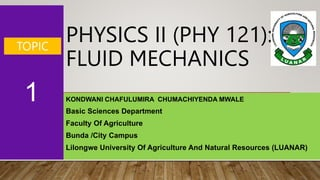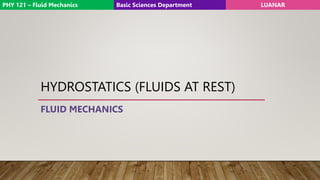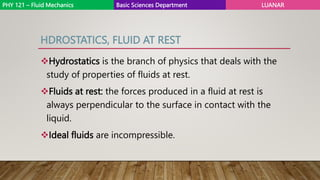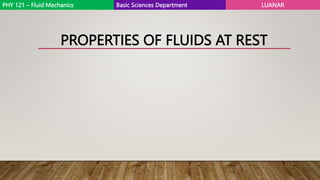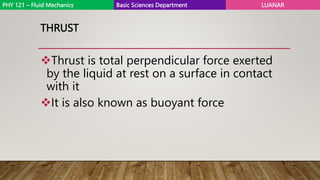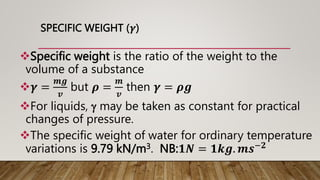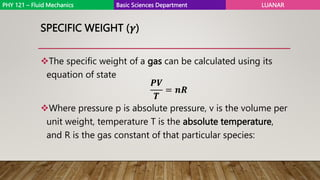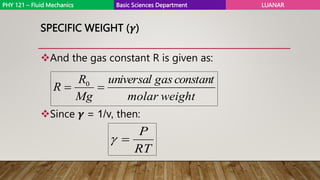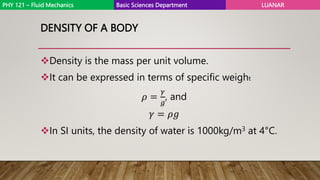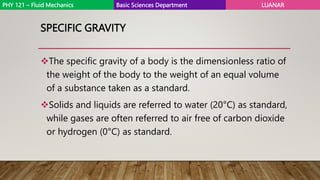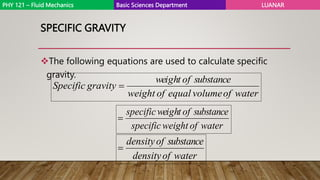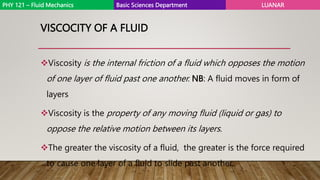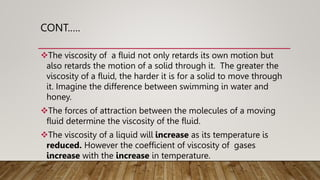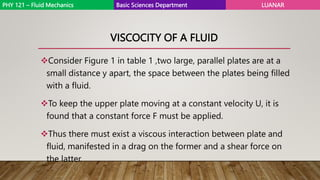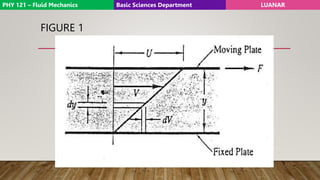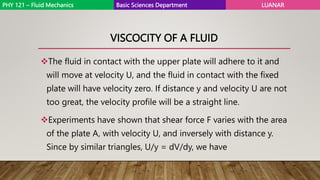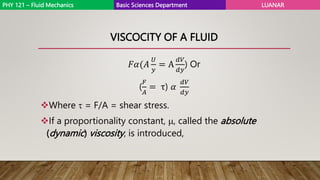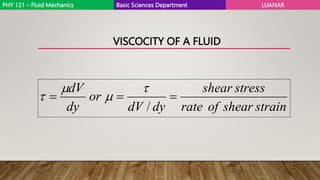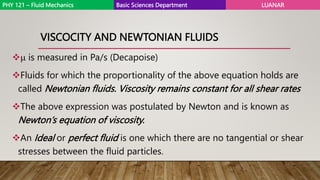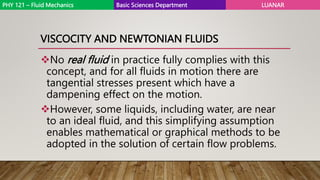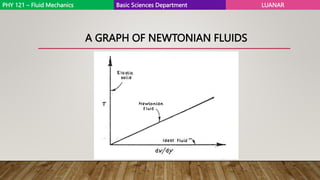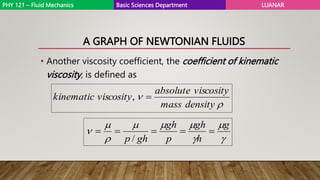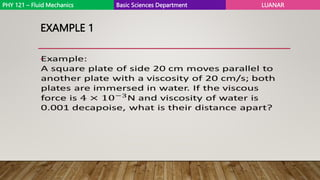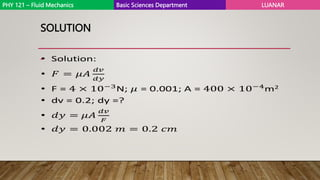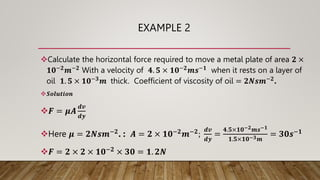1 of 25

### FLUID MECHANICS 2..pptx

• 1. PHYSICS II (PHY 121): FLUID MECHANICS KONDWANI CHAFULUMIRA CHUMACHIYENDA MWALE Basic Sciences Department Faculty Of Agriculture Bunda /City Campus Lilongwe University Of Agriculture And Natural Resources (LUANAR) 1 TOPIC
• 2. HYDROSTATICS (FLUIDS AT REST) FLUID MECHANICS PHY 121 – Fluid Mechanics Basic Sciences Department LUANAR
• 3. HDROSTATICS, FLUID AT REST Hydrostatics is the branch of physics that deals with the study of properties of fluids at rest. Fluids at rest: the forces produced in a fluid at rest is always perpendicular to the surface in contact with the liquid. Ideal fluids are incompressible. PHY 121 – Fluid Mechanics Basic Sciences Department LUANAR
• 4. PROPERTIES OF FLUIDS AT REST PHY 121 – Fluid Mechanics Basic Sciences Department LUANAR
• 5. THRUST Thrust is total perpendicular force exerted by the liquid at rest on a surface in contact with it It is also known as buoyant force PHY 121 – Fluid Mechanics Basic Sciences Department LUANAR
• 6. SPECIFIC WEIGHT (𝜸) Specific weight is the ratio of the weight to the volume of a substance 𝜸 = 𝒎𝒈 𝒗 but 𝝆 = 𝒎 𝒗 then 𝜸 = 𝝆𝒈 For liquids,  may be taken as constant for practical changes of pressure. The specific weight of water for ordinary temperature variations is 9.79 kN/m3. NB:𝟏𝑵 = 𝟏𝒌𝒈. 𝒎𝒔−𝟐
• 7. SPECIFIC WEIGHT (𝜸) The specific weight of a gas can be calculated using its equation of state Where pressure p is absolute pressure, v is the volume per unit weight, temperature T is the absolute temperature, and R is the gas constant of that particular species: 𝑷𝑽 𝑻 = 𝒏𝑹 PHY 121 – Fluid Mechanics Basic Sciences Department LUANAR
• 8. SPECIFIC WEIGHT (𝜸) And the gas constant R is given as: Since 𝜸 = 1/v, then: weight molar t n a t s n o c s a g l a s r e v i n u Mg R R   0 RT P   PHY 121 – Fluid Mechanics Basic Sciences Department LUANAR
• 9. DENSITY OF A BODY Density is the mass per unit volume. It can be expressed in terms of specific weight 𝜌 = 𝛾 𝑔 , and 𝛾 = 𝜌𝑔 In SI units, the density of water is 1000kg/m3 at 4°C. PHY 121 – Fluid Mechanics Basic Sciences Department LUANAR
• 10. SPECIFIC GRAVITY The specific gravity of a body is the dimensionless ratio of the weight of the body to the weight of an equal volume of a substance taken as a standard. Solids and liquids are referred to water (20°C) as standard, while gases are often referred to air free of carbon dioxide or hydrogen (0°C) as standard. PHY 121 – Fluid Mechanics Basic Sciences Department LUANAR
• 11. SPECIFIC GRAVITY The following equations are used to calculate specific gravity. water of volume equal of weight e c n a t s b u s of t h g i e w gravity Specific  water of weight specific e c n a t s b u s of t h g i e w specific  water of density e c n a t s b u s of density  PHY 121 – Fluid Mechanics Basic Sciences Department LUANAR
• 12. VISCOCITY OF A FLUID Viscosity is the internal friction of a fluid which opposes the motion of one layer of fluid past one another. NB: A fluid moves in form of layers Viscosity is the property of any moving fluid (liquid or gas) to oppose the relative motion between its layers. The greater the viscosity of a fluid, the greater is the force required to cause one layer of a fluid to slide past another. PHY 121 – Fluid Mechanics Basic Sciences Department LUANAR
• 13. CONT.…. The viscosity of a fluid not only retards its own motion but also retards the motion of a solid through it. The greater the viscosity of a fluid, the harder it is for a solid to move through it. Imagine the difference between swimming in water and honey. The forces of attraction between the molecules of a moving fluid determine the viscosity of the fluid. The viscosity of a liquid will increase as its temperature is reduced. However the coefficient of viscosity of gases increase with the increase in temperature.
• 14. VISCOCITY OF A FLUID Consider Figure 1 in table 1 ,two large, parallel plates are at a small distance y apart, the space between the plates being filled with a fluid. To keep the upper plate moving at a constant velocity U, it is found that a constant force F must be applied. Thus there must exist a viscous interaction between plate and fluid, manifested in a drag on the former and a shear force on the latter. PHY 121 – Fluid Mechanics Basic Sciences Department LUANAR
• 15. FIGURE 1 PHY 121 – Fluid Mechanics Basic Sciences Department LUANAR
• 16. VISCOCITY OF A FLUID The fluid in contact with the upper plate will adhere to it and will move at velocity U, and the fluid in contact with the fixed plate will have velocity zero. If distance y and velocity U are not too great, the velocity profile will be a straight line. Experiments have shown that shear force F varies with the area of the plate A, with velocity U, and inversely with distance y. Since by similar triangles, U/y = dV/dy, we have PHY 121 – Fluid Mechanics Basic Sciences Department LUANAR
• 17. VISCOCITY OF A FLUID 𝐹𝛼(𝐴 𝑈 𝑦 = A 𝑑𝑉 𝑑𝑦 ) Or ( 𝐹 𝐴 = τ) 𝛼 𝑑𝑉 𝑑𝑦 Where  = F/A = shear stress. If a proportionality constant, , called the absolute (dynamic) viscosity, is introduced, PHY 121 – Fluid Mechanics Basic Sciences Department LUANAR
• 18. VISCOCITY OF A FLUID strain shear of rate stress shear dy dV or dy dV    /     PHY 121 – Fluid Mechanics Basic Sciences Department LUANAR
• 19. VISCOCITY AND NEWTONIAN FLUIDS  is measured in Pa/s (Decapoise) Fluids for which the proportionality of the above equation holds are called Newtonian fluids. Viscosity remains constant for all shear rates The above expression was postulated by Newton and is known as Newton’s equation of viscosity. An Ideal or perfect fluid is one which there are no tangential or shear stresses between the fluid particles. PHY 121 – Fluid Mechanics Basic Sciences Department LUANAR
• 20. VISCOCITY AND NEWTONIAN FLUIDS No real fluid in practice fully complies with this concept, and for all fluids in motion there are tangential stresses present which have a dampening effect on the motion. However, some liquids, including water, are near to an ideal fluid, and this simplifying assumption enables mathematical or graphical methods to be adopted in the solution of certain flow problems. PHY 121 – Fluid Mechanics Basic Sciences Department LUANAR
• 21. A GRAPH OF NEWTONIAN FLUIDS PHY 121 – Fluid Mechanics Basic Sciences Department LUANAR
• 22. A GRAPH OF NEWTONIAN FLUIDS • Another viscosity coefficient, the coefficient of kinematic viscosity, is defined as   density mass osity c vis absolute sity o c vis kinematic  ,          g h gh p gh gh p      / PHY 121 – Fluid Mechanics Basic Sciences Department LUANAR
• 23. EXAMPLE 1 • PHY 121 – Fluid Mechanics Basic Sciences Department LUANAR
• 24. SOLUTION • PHY 121 – Fluid Mechanics Basic Sciences Department LUANAR
• 25. EXAMPLE 2 Calculate the horizontal force required to move a metal plate of area 𝟐 × 𝟏𝟎−𝟐𝒎−𝟐 With a velocity of 𝟒. 𝟓 × 𝟏𝟎−𝟐𝒎𝒔−𝟏 when it rests on a layer of oil 𝟏. 𝟓 × 𝟏𝟎−𝟑𝒎 thick. Coefficient of viscosity of oil = 𝟐𝑵𝒔𝒎−𝟐. 𝑺𝒐𝒍𝒖𝒕𝒊𝒐𝒏 𝑭 = 𝝁𝑨 𝒅𝒗 𝒅𝒚 Here 𝝁 = 𝟐𝑵𝒔𝒎−𝟐 . : 𝑨 = 𝟐 × 𝟏𝟎−𝟐 𝒎−𝟐 ; 𝒅𝒗 𝒅𝒚 = 𝟒.𝟓×𝟏𝟎−𝟐𝒎𝒔−𝟏 𝟏.𝟓×𝟏𝟎−𝟑𝒎 = 𝟑𝟎𝒔−𝟏 𝑭 = 𝟐 × 𝟐 × 𝟏𝟎−𝟐 × 𝟑𝟎 = 𝟏. 𝟐𝑵